# Difference between revisions of "Stochastic matrix"

2010 Mathematics Subject Classification: Primary: 15B51 Secondary: 60J10 [MSN][ZBL]

A stochastic matrix is a square (possibly infinite) matrix $P=[p_{ij}]$ with non-negative elements, for which $$\sum_j p_{ij} = 1 \quad \text{for all i.}$$ The set of all stochastic matrices of order $n$ is the convex hull of the set of $n^n$ stochastic matrices consisting of zeros and ones. Any stochastic matrix $P$ can be considered as the matrix of transition probabilities of a discrete Markov chain $\xi^P(t)$.

The absolute values of the eigenvalues of stochastic matrices do not exceed 1; 1 is an eigenvalue of any stochastic matrix. If a stochastic matrix $P$ is indecomposable (the Markov chain $\xi^P(t)$ has one class of positive states), then 1 is a simple eigenvalue of $P$ (i.e. it has multiplicity 1); in general, the multiplicity of the eigenvalue 1 coincides with the number of classes of positive states of the Markov chain $\xi^P(t)$. If a stochastic matrix is indecomposable and if the class of positive states of the Markov chain has period $d$, then the set of all eigenvalues of $P$, as a set of points in the complex plane, is mapped onto itself by rotation through an angle $2\pi/d$. When $d=1$, the stochastic matrix $P$ and the Markov chain $\xi^P(t)$ are called aperiodic.

The left eigenvectors $\pi = \{\pi_j\}$ of $P$ of finite order, corresponding to the eigenvalue 1: $$\pi_j = \sum_i \pi_i p_{ij} \quad \text{for all j,}$$ and satisfying the conditions $\pi_j \geq 0$, $\sum_j\pi_j = 1$, define the stationary distributions of the Markov chain $\xi^P(t)$; in the case of an indecomposable matrix $P$, the stationary distribution is unique.

Ifis an indecomposable aperiodic stochastic matrix of finite order, then the following limit exists: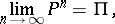(2)

where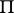is the matrix all rows of which coincide with the vector(see also Markov chain, ergodic; for infinite stochastic matrices, the system of equations (1) may have no non-zero non-negative solutions that satisfy the condition; in this caseis the zero matrix). The rate of convergence in (2) can be estimated by a geometric progression with any exponentthat has absolute value greater than the absolute values of all the eigenvalues ofother than 1.

If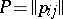is a stochastic matrix of order, then any of its eigenvaluessatisfies the inequality (see [MM]):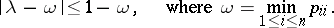The union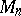of the sets of eigenvalues of all stochastic matrices of orderhas been described (see [Ka]).

A stochastic matrixthat satisfies the extra condition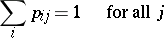is called a doubly-stochastic matrix. The set of doubly-stochastic matrices of orderis the convex hull of the set ofpermutation matrices of order(i.e. doubly-stochastic matrices consisting of zeros and ones). A finite Markov chain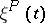with a doubly-stochastic matrixhas the uniform stationary distribution.